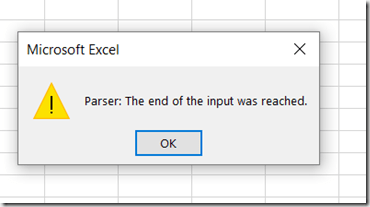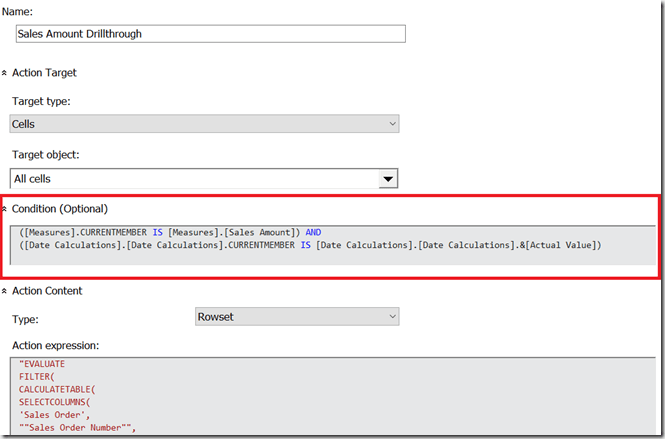# Drillthrough On Calculated Members In SSAS MD 2017 Using DAX Expressions, Part 2

If you read part 1 of this series you probably already have a good idea of how I’m going to use DAX queries in actions to implement drillthrough on calculated members. However, there is one last major problem to solve – and no 100% fool-proof way of solving it.

That problem is that there is a maximum length of (as far as I can tell) 32768 characters for the DAX query that is generated by the action. If you exceed that limit you’ll see an error in Excel when you drillthrough:This is the result of the generated DAX query being truncated before Excel tries to run it. As you can imagine, if you have large dimensions and you’re generating a DAX query with thousands of key values in an IN expression, it’s very easy to hit the maximum query length. What can you do to make this less likely?

Probably the most effective method is to check whether the IN clause will contain all of the possible key values on the dimension (most likely because no attributes from the dimension are used in the query), and if so don’t bother including that dimension in the DAX query. Here’s an example of how this can be done:

```IIF(
COUNT(EXISTING [Date].[Date].[Date].MEMBERS) =
COUNT([Date].[Date].[Date].MEMBERS),
"",
",'Date'[Date.Key0] IN {
" +
GENERATE(EXISTING [Date].[Date].[Date].MEMBERS ,
[Date].[Date].CURRENTMEMBER.PROPERTIES("KEY"), ",")
+ "}
")
```

Another option is to use NonEmpty() to remove any members from the drillthrough that have no data, like so:

```IIF(
COUNT(EXISTING [Date].[Date].[Date].MEMBERS) =
COUNT([Date].[Date].[Date].MEMBERS),
"",
",'Date'[Date.Key0] IN {
" +
GENERATE(EXISTING
NONEMPTY([Date].[Date].[Date].MEMBERS, [Measures].[Sales Amount])
, [Date].[Date].CURRENTMEMBER.PROPERTIES("KEY"), ",")
+ "}
")
```

However, if these techniques (and there may be others) still don’t reduce the length of the query to an acceptable length you will want to try to fail as gracefully as possible and show a more helpful error message than the one above. You can do this with the DAX Error() function, like so:

```IIF(
COUNT(EXISTING [Customer].[Customer].[Customer].MEMBERS) =
COUNT([Customer].[Customer].[Customer].MEMBERS),
"",
",'Customer'[Customer.Key0] IN {
" +
IIF(COUNT(
{EXISTING
NONEMPTY(
[Customer].[Customer].[Customer].MEMBERS,
[Measures].[Sales Amount])}
AS CustomerList)>7000,
"ERROR(
or remove it from your PivotTable"")",
GENERATE(
CustomerList
, [Customer].[Customer].CURRENTMEMBER.PROPERTIES("KEY"), ",")
)
+ "}
")
```

In this example, if the resulting DAX IN expression for the Customer dimension will have more than 7000 keys in it, the entire DAX query returns a custom error message instead:Once again this is a far from perfect solution – I would have liked to test the total number of characters in the query, but if you do that you have to write the expression twice, once in the first parameter of IIF() and once in one of the results, and that would be horrible. My gut feeling is that you should only use this technique on dimensions with a large number of members on the key attribute.

Putting this all together, for a simple cube based on data from Adventure Works with three dimensions (Date, Product and Customer) here’s what a complete Action Expression for a regular measure might look like:

```"EVALUATE
FILTER(
CALCULATETABLE(
SELECTCOLUMNS(
'Sales Order',
""Sales Order Number"",
'Sales Order'[Sales Order Number],
""Sales Order Line Number"",
'Sales Order'[Sales Order Line Number],
""Sales Amount"",
[Sales Amount])
" +
IIF(
COUNT(EXISTING [Date].[Date].[Date].MEMBERS) =
COUNT([Date].[Date].[Date].MEMBERS),
"",
",'Date'[Date.Key0] IN {
" +
GENERATE(EXISTING
NONEMPTY([Date].[Date].[Date].MEMBERS,
[Measures].[Sales Amount])
, [Date].[Date].CURRENTMEMBER.PROPERTIES("KEY"), ",")
+ "}
") +
IIF(
COUNT(EXISTING [Customer].[Customer].[Customer].MEMBERS) =
COUNT([Customer].[Customer].[Customer].MEMBERS),
"",
",'Customer'[Customer.Key0] IN {
" +
IIF(
COUNT(
{EXISTING NONEMPTY([Customer].[Customer].[Customer].MEMBERS,
[Measures].[Sales Amount])} AS CustomerList)>7000,
or remove it from your PivotTable"")",
GENERATE(
CustomerList
, [Customer].[Customer].CURRENTMEMBER.PROPERTIES("KEY"), ",")
)
+ "}
") +
IIF(
COUNT(EXISTING [Product].[Product].[Product].MEMBERS) =
COUNT([Product].[Product].[Product].MEMBERS),
"",
",'Product'[Product.Key0] IN {
" +
GENERATE(EXISTING
NONEMPTY([Product].[Product].[Product].MEMBERS,
[Measures].[Sales Amount])
, [Product].[Product].CURRENTMEMBER.PROPERTIES("KEY"), ",")
+ "}
") +
"),
[Sales Amount]>0)"
```

What about a calculated measure though? Assuming that  you have a time utility/date tool/shell dimension, you can use a Condition expression on the action above to make sure it only gets executed for the member on the time utility dimension that contains the non-calculated values, in this case called [Actual Value]:

```([Measures].CURRENTMEMBER IS [Measures].[Sales Amount]) AND
([Date Calculations].[Date Calculations].CURRENTMEMBER IS
[Date Calculations].[Date Calculations].&[Actual Value])
```Now, let’s say there is a calculated member on the time utility dimension that contains a year-to-date calculation with the following definition:

```CREATE MEMBER
CURRENTCUBE.[Date Calculations].[Date Calculations].[Year-To-Date]
AS
AGGREGATE(
PERIODSTODATE(
[Date].[Calendar].[Year],
[Date].[Calendar].CURRENTMEMBER),
[Date Calculations].[Date Calculations].&[Actual Value]);
```

You can create a new action that has a Condition expression as follows that restricts it to the year-to-date calculation:

```([Measures].CURRENTMEMBER IS [Measures].[Sales Amount]) AND
([Date Calculations].[Date Calculations].CURRENTMEMBER IS
[Date Calculations].[Date Calculations].[Year-To-Date])
```

Now, the final problem to solve is to generate a DAX query that returns all of the Sales Orders from the beginning of the current year up to and including the selected date – the Sales Orders that a user would expect to see when they drilled through on the year-to-date calculated member above.

Here’s a modified MDX expression for the Date dimension that returns a DAX expression that finds all of the dates associated with the current selection on the Date dimension, finds the maximum date, then filters the drillthrough DAX query by all dates from the beginning of the current calendar year up to and including the maximum date:

```IIF(
COUNT(EXISTING [Date].[Date].[Date].MEMBERS) =
COUNT([Date].[Date].[Date].MEMBERS),
"",
", VAR FilteredDates = FILTER('Date', 'Date'[Date.Key0] IN {
" +
GENERATE(
EXISTING
NONEMPTY([Date].[Date].[Date].MEMBERS,[Measures].[Sales Amount]) ,
[Date].[Date].CURRENTMEMBER.PROPERTIES("KEY"), ",")
+ "})
VAR MaxDate = MAXX(FilteredDates, 'Date'[Date.Key0])
VAR MaxYear = MAXX(FilteredDates, 'Date'[Year])
RETURN
FILTER('Date', 'Date'[Date.Key0]<=MaxDate && 'Date'[Year]=MaxYear)
")
```

Here’s the complete MDX expression for the year-to-date drillthrough:

```"EVALUATE
FILTER(
CALCULATETABLE(
SELECTCOLUMNS(
'Sales Order',
""Sales Order Number"",
'Sales Order'[Sales Order Number],
""Sales Order Line Number"",
'Sales Order'[Sales Order Line Number],
""Sales Amount"",
[Sales Amount])
" +
IIF(
COUNT(EXISTING [Date].[Date].[Date].MEMBERS) =
COUNT([Date].[Date].[Date].MEMBERS),
"",
", VAR FilteredDates = FILTER('Date', 'Date'[Date.Key0] IN {
" +
GENERATE(
EXISTING
NONEMPTY([Date].[Date].[Date].MEMBERS,[Measures].[Sales Amount]) ,
[Date].[Date].CURRENTMEMBER.PROPERTIES("KEY"), ",")
+ "})
VAR MaxDate = MAXX(FilteredDates, 'Date'[Date.Key0])
VAR MaxYear = MAXX(FilteredDates, 'Date'[Year])
RETURN
FILTER('Date', 'Date'[Date.Key0]<=MaxDate && 'Date'[Year]=MaxYear)
") +
IIF(
COUNT(EXISTING [Customer].[Customer].[Customer].MEMBERS) =
COUNT([Customer].[Customer].[Customer].MEMBERS),
"",
",'Customer'[Customer.Key0] IN {
" +
IIF(COUNT({
EXISTING
NONEMPTY([Customer].[Customer].[Customer].MEMBERS,
[Measures].[Sales Amount])} AS CustomerList)>7000,
GENERATE(
CustomerList
, [Customer].[Customer].CURRENTMEMBER.PROPERTIES("KEY"), ",")
)
+ "}
") +
IIF(
COUNT(EXISTING [Product].[Product].[Product].MEMBERS) =
COUNT([Product].[Product].[Product].MEMBERS),
"",
",'Product'[Product.Key0] IN {
" +
GENERATE(EXISTING
NONEMPTY([Product].[Product].[Product].MEMBERS,
[Measures].[Sales Amount])
, [Product].[Product].CURRENTMEMBER.PROPERTIES("KEY"), ",")
+ "}
") +
"),
[Sales Amount]>0)"
```

I warned you it was going to be complicated, didn’t I? You can download a SSAS MD 2017 .abf backup file containing the sample database and the two actions here.

### 2 responses

1.Ron Baerson says:

What would be the syntax to add in another dimension? I’ve tried using Related(‘Date'[Date]) for example and keep getting an error message that the table is not related.

•Chris Webb says:

Is there a relationship between the tables? Related() should work but only if a relationship is present between the table used in the first parameter of SelectColumns() and your Date table.

This site uses Akismet to reduce spam. Learn how your comment data is processed.